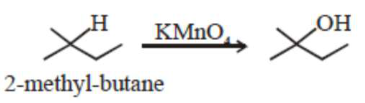# Given below are two statements:

Question:

Given below are two statements:

Statement-I : 2 -methylbutane on oxidation with $\mathrm{KMnO}_{4}$ gives 2 methylbutan- $2-o 1$.

Statement-II : n-alkanes can be easily oxidised to corresponding alcohol with $\mathrm{KMnO}_{4}$. Choose the correct option :

1. Both statement $\mathrm{I}$ and statement $\Pi$ are correct

2. Both statement I and statement II are incorrect

3.  Statement I is correct but Statement II is incorrect

4. Statement I is incorrect but Statement II is correct

Correct Option: , 3

Solution:

Alkane are very less reactive, tertiary hydrogen can oxidise to alcohal with $\mathrm{KMnO}_{4}$